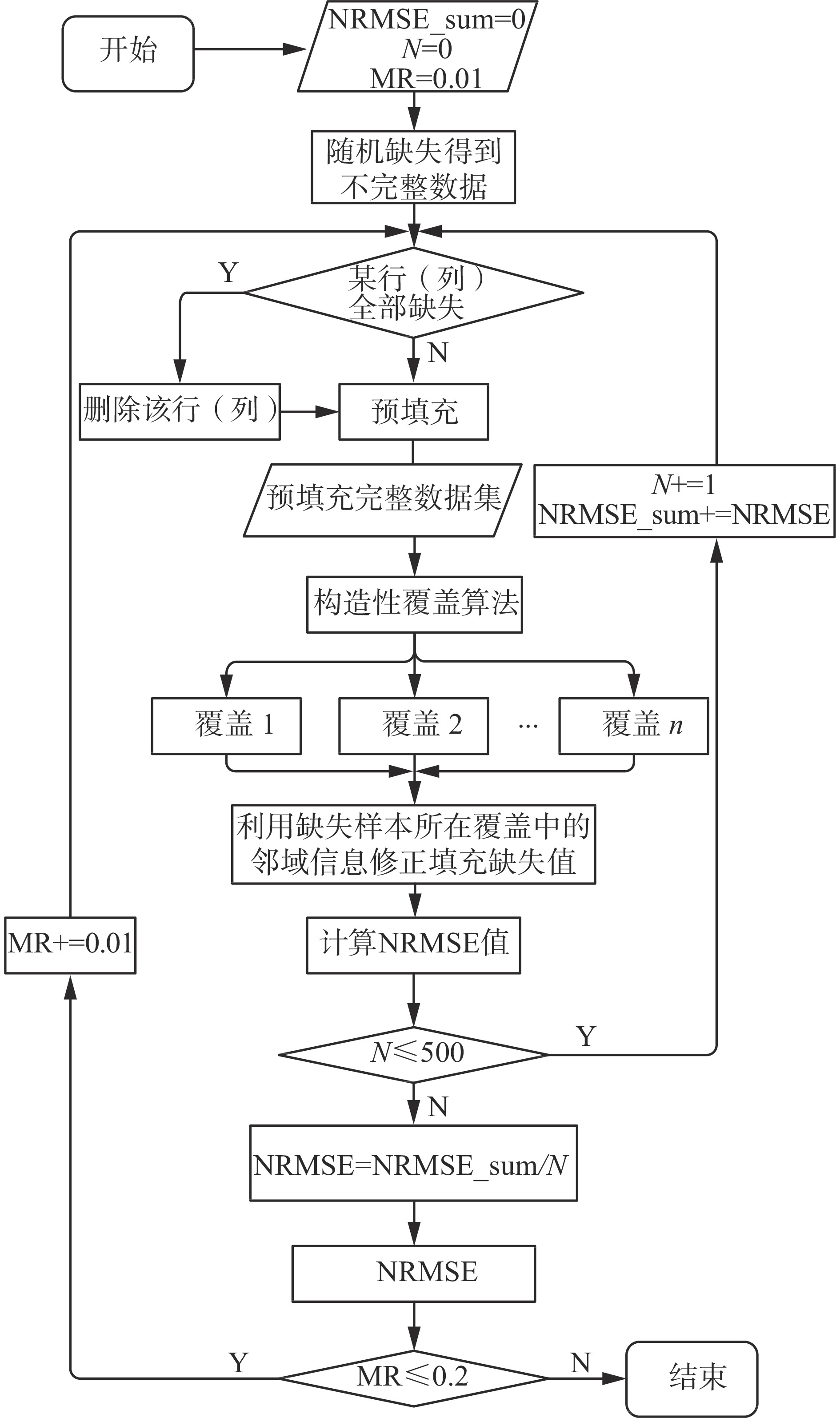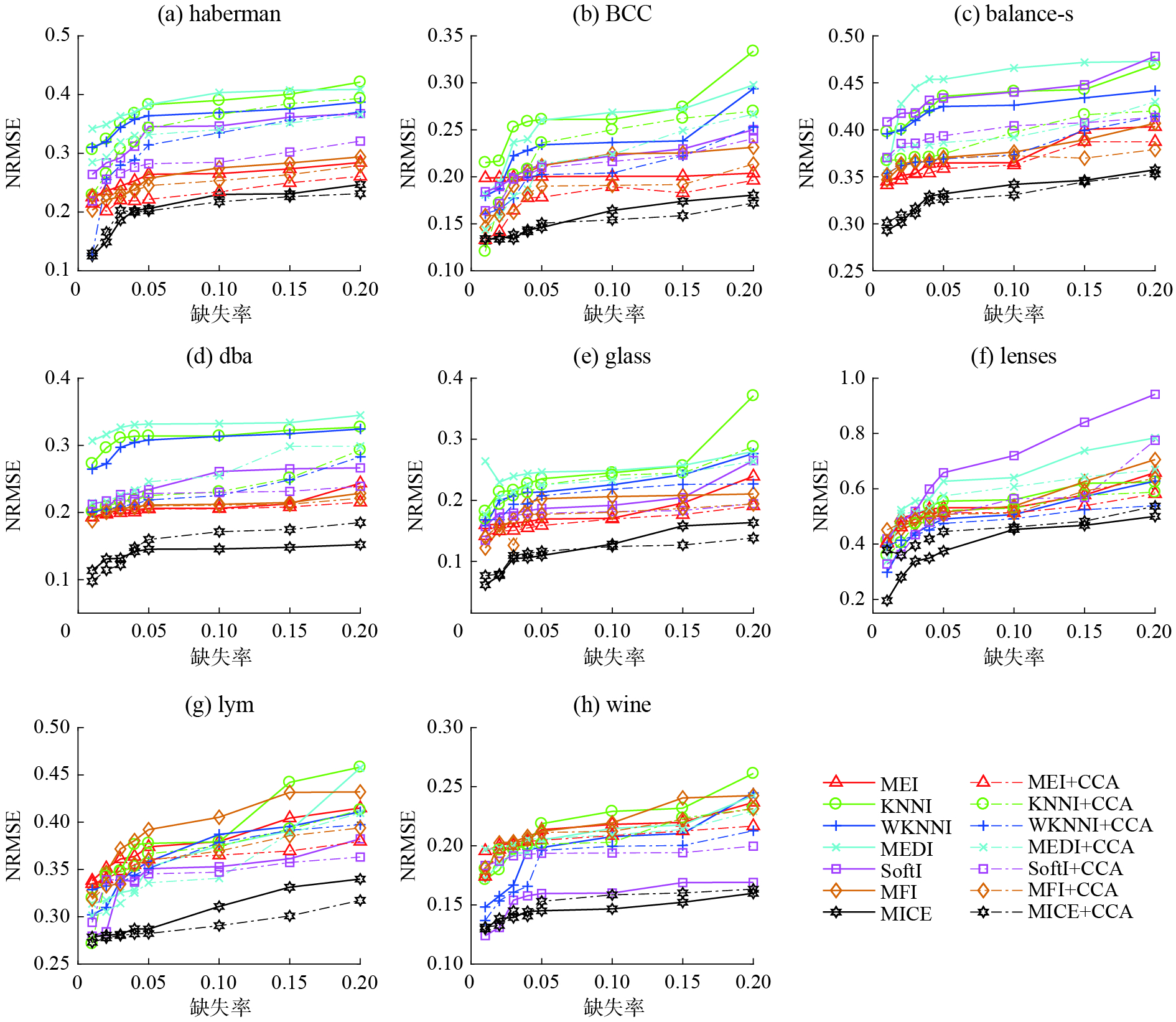﻿ 构造性覆盖下不完整数据修正填充方法
«上一篇文章快速检索 高级检索

 智能系统学报2019, Vol. 14Issue (6): 1225-1232  DOI: 10.11992/tis.2019060150

### 引用本文YAN Yuanting, WU Yaya, ZHAO Shu, et al. Improving missing data recovery with a constructive covering algorithm[J]. CAAI Transactions on Intelligent Systems, 2019, 14(6): 1225-1232. DOI: 10.11992/tis.201906015.### 文章历史

Improving missing data recovery with a constructive covering algorithm
YAN Yuanting , WU Yaya , ZHAO Shu , ZHANG YanpingSchool of Computer Science and Technology, Anhui University, Hefei 230601, China
Abstract: Incomplete data processing is one of the most active avenues in the fields of data mining, machine learning, etc. Missing value imputation is the mainstream method used to deal with incomplete data. At present, most existing missing value imputation methods utilize relevant techniques in the field of statistics and machine learning to analyze surplus information from original data to replace the missing attributes with plausible values. Missing value imputation can be roughly divided into single imputation and multiple imputation, which have their own advantages in different scenarios. However, there are few methods that can further consider neighborhood information in the spatial distribution of samples and modify the filling results of missing values. In view of this, this paper proposes a new framework that can be widely used in many existing imputation methods to enhance the imputation effect of existing methods. It is composed of three modules, called pre-filling, spatial neighborhood information mining, and modification of the results of pre-filling separately. In this paper, seven existing imputation methods were evaluated on eight UCI datasets. Experimental results verified the validity and robustness of the framework proposed in this paper.
Key words: incomplete data    missing value imputation    neighborhood information    data-mining    machine learning    imputation method    single imputation    multiple imputation

1 相关工作 1.1 缺失机制和缺失模式

1.2 构造性覆盖算法

2 基于空间邻域信息的修正填充方法 2.1 预填充

 ${\rm IND} = \{ (i,j)|x_i^{(j)} = NaN\}$ (1)

1) 均值填充(MEI)

 $x_i^{(j)} = \mathop \sum \limits_{k \in I({\rm comp})} \frac{{x_k^{(j)}}}{{{n_{|I({\rm comp})|}}}}$ (2)

2) 中值填充(MEDI)

$\varOmega = \left\{ {x_k^{(j)}{{|k}} \in I({\rm comp})} \right\}$ ${\varOmega ^*}$ 表示集合 $\varOmega$ 中所有元素的升序存放； $|\varOmega | = |{\varOmega ^{\rm{*}}}{\rm{|}} = n$ ；则：

 $x_i^j = \left\{ {\begin{array}{*{20}{c}} {\!\!\!\!\!\!\!\!\!\!\!\!\!\!\!\!\!\!\!\!\!\!\!\!\!\!\!\!\!\!\!\!\!\!\!\!\!\!\!\!{\varOmega ^*}_{\left[ {n + 1/2} \right]}},&{n\;od 2 \ne 0}\\ {\left( {{\varOmega ^*}_{\left[ {n/2} \right]} + {\varOmega ^*}_{\left[ {n + 1/2} \right]}} \right)/2},&{n\;od 2 = 0} \end{array}} \right.$ (3)

3) KNNI

 $d_i^t = {\rm dist} \left( {x_i,x_t} \right) = \sqrt {\mathop \sum \limits_{p = 1}^n {{\left( {x_i^p - x_t^p} \right)}^2}}$ (4)
 $x_i^j = \left( {\mathop \sum \limits_{\forall {x_t} \in {N_i}} x_t^j} \right)/\left| {{N_i}} \right|,\;\;\;\;x_t^j \ne {\rm NaN}$ (5)

4) WKNNI

 $x_i^j = \mathop \sum \limits_{\forall {x_t} \in {N_i}} x_t^j{\omega _{t}}\;,\;\;\;\;{\omega _t} = \frac{{1/d_i^t}}{{\mathop \sum \limits_{t = 1}^k 1/d_i^t}}$ (6)

5) Soft-impute(SoftI)：通过对SVD分解的迭代软阈值处理来填充不完整数据。

6) Matrix-Factorization-impute(MFI)：将不完整数据用矩阵形式表示并直接将其分解为低秩的UV，然后对U中的元素采用L1稀疏惩罚，对V中的元素采用L2稀疏惩罚，通过梯度下降法求解。

7) MICE：利用链式方程实现多重填充。

2.2 挖掘空间邻域信息

1)通过式(2)变换将 ${\rm DS}{_c}$ 中的样本点投射到 ${S^{n + 1}}$ 球面上并使得投影后的样本向量等长。

 ${\rm{}}T:{\rm DS}{_c} \to {S^{n + 1}},T\left( x \right) = \left( {x,\sqrt {{R^2} - {{\left| x \right|}^2}} } \right)$ (7)

2)随机选取一个未被标记的样本 $x_k$ 作为覆盖中心并计算覆盖半径R

 $< x_k,x_i > x_{k}^{\left( 1 \right)}x_{i}^{\left( 1 \right)} + \cdots + x_{k}^{\left( {n + 1} \right)}x_{i}^{\left( {n + 1} \right)},\;\;\;i \in \left\{ {1,2, \cdots, m} \right\}$ (8)
 ${d_1} = \mathop {\max }\limits_{y_k{ \ne }y_i} \left\{ {\left\langle {x_k,x_i} \right\rangle } \right\},\;\;\;i \in \left\{ {1,2, \cdots, m} \right\}$ (9)
 ${d_2} = \mathop {\min }\limits_{y_k = y{{_i}}} \left\{ {\left\langle {x_k,x_i} \right\rangle {\rm{|}}\left\langle {x_k,x_i} \right\rangle > {d_1}} \right\},\;\;\;i \in \left\{ {1,2, \cdots, m} \right\}$ (10)
 $R = ({d_1} + {d_2})/2$ (11)

3)构造一个以 ${x_{_k}}$ 为球形领域的中心，R为半径的球形领域 $C_v$ ，其中 $C_v^k$ 表示第v类样本的第k个覆盖，并将该领域内的所有样本都标记成“已学习”。若全部已被标记则会得到一组覆盖集合 $C = \{ C_1^1,C_1^2, \cdots ,C_1^{{k_1}},C_2^1,C_2^2, \cdots ,C_2^{{k_2}}, \cdots ,C_i^1, \cdots \}$ ，否则返回2)。

2.3 利用空间邻域信息修正预填充结果

${C_1}$ ${C_2}$ 为经过2.2节所得到的两类样本的覆盖集合为

 ${C_1} = \left\{ {C_1^1,C_1^2, \cdots ,C_1^{{k_1}}} \right\};{C_2} = \left\{ {C_2^1,C_2^2, \cdots ,C_2^{{k_2}}} \right\}$ (12)

 $x_i^{\left( j \right)} = \left\{ {\begin{array}{*{20}{l}} {(\mathop \sum \limits_{t = 1}^{\left| \psi \right|} {{(x_s^{\left( j \right)})}_t})/\left| \psi \right|},&{\left| \psi \right| \ne 0}\\ {x_p^{\left( j \right)}},&\!\!\!\!\!\!\!\!\!\!\!\!\!\!\!\!\!\!\!\!\!\!\!\!\!\!\!\!\!\!\!\!\!\!{\left| \psi \right| = 0} \end{array}} \right.$ (13)
 $\psi = \left\{ x_{s}^{(j)}| {x_s} \in \left\{ C_{v}^k - x_i \right\} \wedge (s,j) \notin {\rm IND} \right\}$ (14)Download: 图 1 算法流程图 Fig. 1 The flow chart of the proposed method
3 实验与分析 3.1 实验设计

3.2 实验数据集表 1 数据集简介 Tab.1 The introduction of the datasets
3.3 不完整数据填充性能的评价标准

 ${\rm{NRMSE}} = \frac{{\sqrt {{\rm mean}{{({x_{\rm guess}} - {x_{\rm ori}})}^2}} }}{{{\rm std}\left( {{x_{\rm ori}}} \right)}}$ (15)

3.4 实验结果与分析Download: 图 2 7组填充方法在8个数据集上以及在不同缺失率下对应的NRMSE值 Fig. 2 The NRMSE values corresponding to 7 groups of imputation methods on 8 datasets and at different missing ratesDownload: 图 3 7组现有填充方法经修正后的填充效果提升图 Fig. 3 The improvement of the imputation effect by using proposed method
4 结束语

  LARRAÑAGA P, CALVO B, SANTANA R, et al. Machine learning in bioinformatics[J]. Briefings in bioinformatics, 2006, 7(1): 86-112. DOI:10.1093/bib/bbk007 (0)  HARPER P R. A review and comparison of classification algorithms for medical decision making[J]. Health policy, 2005, 71(3): 315-331. DOI:10.1016/j.healthpol.2004.05.002 (0)  SEBASTIANI F. Machine learning in automated text categorization[J]. ACM computing surveys, 2002, 34(1): 1-47. DOI:10.1145/505282.505283 (0)  KONG S G, HEO J, ABIDI B R, et al. Recent advances in visual and infrared face recognition—a review[J]. Computer vision and image understanding, 2005, 97(1): 103-135. DOI:10.1016/j.cviu.2004.04.001 (0)  FU Xiao, REN Yinzi, YANG Guiqiu, et al. A computational model for heart failure stratification[C]//Proceedings of 2011 IEEE Computing in Cardiology. Hangzhou, China, 2011: 385–388. (0)  FIALHO A S, KAYMAK U, ALMEIDA R J, et al. Probabilistic fuzzy prediction of mortality in intensive care units[C]//Proceedings of 2012 IEEE International Conference on Fuzzy Systems. Brisbane, Australia, 2012: 1–8. (0)  AITTOKALLIO T. Dealing with missing values in large-scale studies: microarray data imputation and beyond[J]. Briefings in bioinformatics, 2010, 11(2): 253-264. DOI:10.1093/bib/bbp059 (0)  DE SOUTO M C P, JASKOWIAK P A, COSTA I G. Impact of missing data imputation methods on gene expression clustering and classification[J]. BMC bioinformatics, 2015, 16: 64. DOI:10.1186/s12859-015-0494-3 (0)  LIU Siyuan, CHEN Lei, NI L M. Anomaly detection from incomplete data[J]. ACM transactions on knowledge discovery from data, 2014, 9(2): 11. (0)  LIU Ji, MUSIALSKI P, WONKA P, et al. Tensor completion for estimating missing values in visual data[J]. IEEE transactions on pattern analysis and machine intelligence, 2013, 35(1): 208-220. DOI:10.1109/TPAMI.2012.39 (0)  LAKSHMINARAYAN K, HARP S A, SAMAD T. Imputation of missing data in industrial databases[J]. Applied intelligence, 1999, 11(3): 259-275. DOI:10.1023/A:1008334909089 (0)  SONG Qinhao, SHEPPERD M, CHEN Xiangru, et al. Can k-NN imputation improve the performance of C4.5 with small software project data sets? A comparative evaluation[J]. Journal of systems and software, 2008, 81(12): 2361-2370. DOI:10.1016/j.jss.2008.05.008 (0)  DONDERS A R T, VAN DER HEIJDEN G J M G, STIJNEN T, et al. Review: a gentle introduction to imputation of missing values[J]. Journal of clinical epidemiology, 2006, 59(10): 1087-1091. DOI:10.1016/j.jclinepi.2006.01.014 (0)  TROYANSKAYA O, CANTOR M, SHERLOCK G, et al. Missing value estimation methods for DNA microarrays[J]. Bioinformatics, 2001, 17(6): 520-525. DOI:10.1093/bioinformatics/17.6.520 (0)  KEERIN P, KURUTACH W, BOONGOEN T. Cluster-based KNN missing value imputation for DNA microarray data[C]//Proceedings of 2012 IEEE International Conference on Systems, Man, and Cybernetics. Seoul, South Korea, 2012: 445-450. (0)  VAN BUUREN S, GROOTHUIS-OUDSHOORN K. Mice: Multivariate imputation by chained equations in R[J]. Journal of statistical software, 2011, 45(3): 75765. (0)  GEBREGZIABHER M, DESANTIS S M. Latent class based multiple imputation approach for missing categorical data[J]. Journal of statistical planning and inference, 2010, 140(11): 3252-3262. DOI:10.1016/j.jspi.2010.04.020 (0)  VERMUNT J K, VAN GINKEL J R, VAN DER ARK L A, et al. 9. Multiple imputation of incomplete categorical data using latent class analysis[J]. Sociological methodology, 2008, 38(1): 369-397. DOI:10.1111/j.1467-9531.2008.00202.x (0)  TOUTENBURG H. Rubin, D.B.: multiple imputation for nonresponse in surveys[J]. Statistical papers, 1990, 31(1): 180. DOI:10.1007/BF02924688 (0)  SIM J M, KWON O, LEE K C. Adaptive pairing of classifier and imputation methods based on the characteristics of missing values in data sets[J]. Expert systems with applications, 2016, 46: 485-493. DOI:10.1016/j.eswa.2015.11.004 (0)  张铃, 张钹. M-P神经元模型的几何意义及其应用[J]. 软件学报, 1998, 9(5): 334-338. ZHANG Ling, ZHANG Bo. A geometrical representation of M-P neural model and its applications[J]. Journal of software, 1998, 9(5): 334-338. (0)  张燕平, 张铃. 机器学习理论与算法[M]. 北京: 科学出版社, 2012: 56–66. (0)  JÖRNSTEN R, WANG Huiyu, WELSH W J, et al. DNA microarray data imputation and significance analysis of differential expression[J]. Bioinformatics, 2005, 21(22): 4155-4161. DOI:10.1093/bioinformatics/bti638 (0)  MAZUMDER R, HASTIE T, TIBSHIRANI R. Spectral regularization algorithms for learning large incomplete matrices[J]. The journal of machine learning research, 2010, 11: 2287-2322. (0)  RANJBAR M, MORADI P, AZAMI M, et al. An imputation-based matrix factorization method for improving accuracy of collaborative filtering systems[J]. Engineering applications of artificial intelligence, 2015, 46: 58-66. DOI:10.1016/j.engappai.2015.08.010 (0)